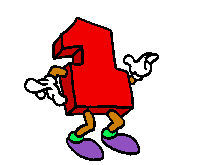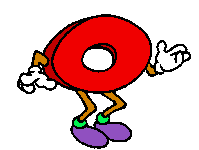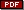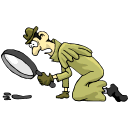Mathwire.com
New on Mathwire.com:   February 2007# 100th Day of School Activities

Numbers: ARG Animation

This page is a collection of math activities that celebrate the 100th day of school. It includes handouts and directions for problem solving, math-literature titles, classroom pictures and links to additional activities on the internet.Added on 2/25/07: Open-ended Assessments contain many pairs of assessments so that one problem may be used for instruction and the other for student independent solving.

•Snowball Piles asks students to look for a pattern and use that pattern to predict how many snowballs they will need to build additional rows.
•Math Pattern requires students to identify the pattern, extend the pattern, classify the pattern, and then describe the pattern in words.
•Shape Pattern requires students to identify the pattern, extend the pattern, classify the pattern, and then describe the pattern in words.
•Flowers uses a function table to organize data so that students can see the rule and use it to extend the table.
•Stickers uses a function table to organize data so that students can see the rule and use it to extend the table.
•Boardwalk Games uses a horizontal table to organize data. Students must write a rule and use it to complete the table. They must also explain how they know their rule and answers are correct.
•Fishing uses a horizontal table to organize data. Students must write a rule and use it to complete the table. They must also explain how they know their rule and answers are correct.

•Totem Pole shows a diagram of Kate's pattern block totem pole and asks students to tell how many of each block she will need to build the totem pole and how much it will cost, given the price of each block.
•Robot Man shows a diagram of Juan's pattern block robot and asks students to tell how many of each block he will need to build the robot and how much it will cost, given the price of each foam block.
•Symmetry Puzzle requires students to complete the design so that the dotted line is a line of symmetry. Students must explain how they know their design is symmetrical.
•Spaceship Puzzle requires students to complete the design so that the dotted line is a line of symmetry. Students must explain how they know their design is symmetrical.